﻿ PipeLay > Technical Notes > Technical Note 6 - Dean's Stream Function

# Technical Note 6 - Dean's Stream Function

Navigation:  PipeLay > Technical Notes >

# Technical Note 6 - Dean's Stream Function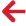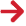## Overview

This technical note describes the implementation of Dean’s stream function in PipeLay. Fourier series wave theory is first outlined, and then the vessel response to the stream function is described. Each of the three vessel motion options – Multiple Order, Equivalent Airy Wave and Superposition of Harmonics – are discussed in turn.

## Introduction

Stream function theory, a non-linear wave theory related to that of Stokes, was developed by Dean in order to examine fully non-linear waves numerically. PipeLay uses a version of Dean’s stream function known as Fenton’s Fourier series theory to compute the wave kinematics for a given set of wave parameters. This method eliminates the need to use tabular or graphical presentations of the solutions in order to solve the stream function equation and is an efficient and accurate solution method.

## Fourier Series Wave Theory

Fourier series wave theory derives its name from an approximate solution to the governing wave equation using a Fourier cosine series in θ, as follows: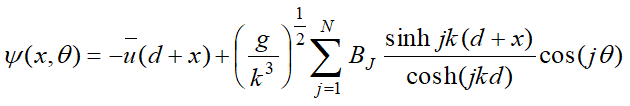(TN.6.1)

 ψ = Stream Function ū = Mean value of horizontal fluid velocity for a constant value of x, over one wavelength d = Water depth x = Height above the Mean Water Line g = Gravity k = Wavenumber BJ = Set of dimensionless Fourier coefficients N = Order of the stream function. This is a measure of how non-linear the  wave is. In deep water the order can be relatively low (between 3 and 5), while in very shallow water the order can be as high as 30.

This formulation for the stream function, combined with the dynamic and kinematic free surface boundary conditions and the dispersion relationship are used to set up a system of complex non-linear equations.  The unknown variables in the stream function equation can then be calculated for any given set of wave height, wave period, water depth and underlying current velocity. The wave kinematics (surface elevation, water particle velocities and accelerations etc.) are then derived from the solution of the governing equation.

## Vessel Response to Stream Function Wave

PipeLay provides three options for calculating the response of a vessel to a Dean’s Stream Function wave, when that response is defined in terms of RAOs in the various degrees of freedom.  These are known as Multiple Order, Equivalent Airy Wave and Superposition of Harmonics.

The water surface elevation at any point in the wave field is found using the following equation: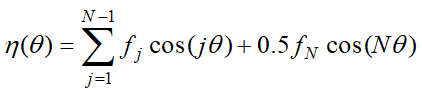(TN.6.2)

Where: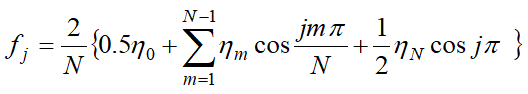(TN.6.3)

and

θ        = ks - ωt

s        = Horizontal distance from vertical axis Y=Z=0 to point in question

= ycosϕ  + zsinϕ

y        = Horizontal distance from vertical axis to point in global Y direction

z         = Horizontal distance from vertical axis to point in global Z direction

ϕ         = Wave direction relative to global Y

ω         = Wave circular frequency  = 2π/T

T        = Wave period

t         = Present solution time.

## Vessel Motions – Multiple Order

The high frequency response Ȓi of the vessel reference point in local degree of freedom i to a Dean’s Stream Function wave when you nominate the Multiple Order option is defined by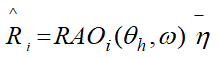(TN.6.4)

where: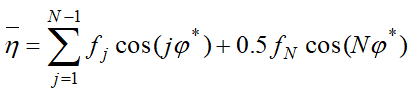(TN.6.5)

and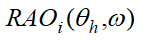= vessel RAO in degree of freedom i, which is a function of wave heading θhand wave frequency ω f = surface elevation function as defined in Equation (TN.6.3)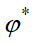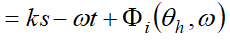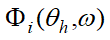= vessel phase angle in degree of freedom i, which is also a function of θh and ω.

Remaining symbols are as previously. This option gives a vessel response of order corresponding to the order of expansion of the Fourier Series.

## Vessel Motions – Equivalent Airy Wave

This option is provided to allow you to specify a sinusoidal vessel response to the multiple order stream function wave. To invoke this facility you specify an equivalent Airy wave amplitude when you are inputting the Stream Function data; you can optionally specify an equivalent wave period. What then happens is the following. The Dean’s Stream Function wave height, period and direction are used in the normal way in calculating water particle kinematics and ultimately wave forces. The equivalent Airy wave parameters on the other hand are combined with the vessel RAOs and phase angles to calculate vessel motions. This will ensure a sinusoidal response.

You can specify an equivalent Airy wave amplitude without inputting a corresponding period, in which case the Dean’s Stream Function wave period is used by default.

The high frequency response Ȓi of the vessel reference point in local degree of freedom i to a Dean’s Stream Function wave when you nominate the Equivalent Airy Wave option is defined by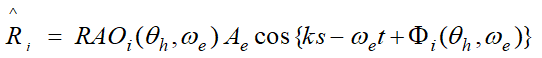(TN.6.6)

where: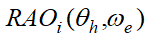= Vessel RAO in degree of freedom i, which is a function of wave heading θh and equivalent wave frequency ωe;

ωe = equivalent Airy wave circular frequency =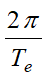Te= period of equivalent Airy wave

Ae= amplitude of equivalent Airy wave, and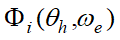= vessel phase angle in degree of freedom i, which is also a function of θh and ωe

Remaining symbols are as previously.

## Vessel Motions – Superposition of Harmonics

The high frequency response Ȓi of the vessel reference point in local degree of freedom i to a Dean’s Stream Function wave when you nominate the Superposition of Harmonics option is defined by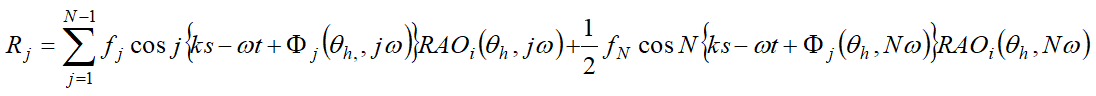(TN.6.7)

where: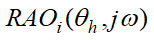= Vessel RAO in degree of freedom i, which is a function of wave heading θh and equivalent wave frequency jω, and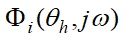= vessel phase angle in degree of freedom i, which is also a function of θh and jω

f = surface elevation function as defined in Equation (TN.6.3)

Remaining symbols are as previously.

With this option, the vessel response is a summation of N components, which correspond to the “components” in the expression for water surface elevation in Equation (TN.6.2). RAO values and phase angles corresponding to each component in Equation (TN.6.2) are extracted from the user-specified data in the usual way, and the vessel response is found by summing over the N terms in a similar manner as would be used in a random sea. Obviously for this exercise to be meaningful RAOs and phase angles must be defined over the full range of circular frequencies from ω to Nω.